## Polyhedral Formula

A formula relating the number of Vertices, Faces, and Edges of a Polyhedron (or Polygon). It was discovered independently by Eulerand Descartes,so it is also known as the Descartes-Euler Polyhedral Formula. The polyhedron need not be Convex, but the Formula does not hold for Stellated Polyhedra.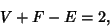(1)

where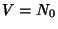is the number of Vertices,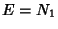is the number of Edges, and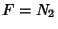is the number of Faces. For a proof, see Courant and Robbins (1978, pp. 239-240). The Formula can be generalized to-D Polytopes.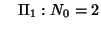(2)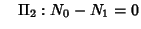(3)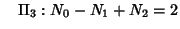(4)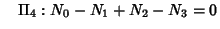(5)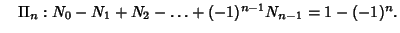(6)
For a proof of this, see Coxeter (1973, pp. 166-171).

References

Beyer, W. H. (Ed.) CRC Standard Mathematical Tables, 28th ed. Boca Raton, FL: CRC Press, p. 128, 1987.

Courant, R. and Robbins, H. What is Mathematics?: An Elementary Approach to Ideas and Methods. Oxford, England: Oxford University Press, 1978.

Coxeter, H. S. M. Regular Polytopes, 3rd ed. New York: Dover, 1973.